Fourier Series Coefficients using Mathematica's FourierSeries package: Preparing the Input Function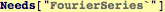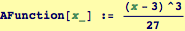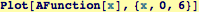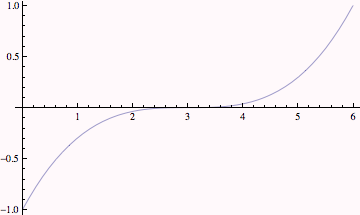Mathematica's Fourier Series functions are defined for function that are periodic in the domain x ∈ (-1/2,1/2).  So we need to map the periodic functions to this domain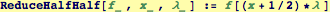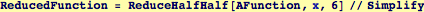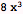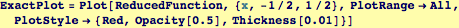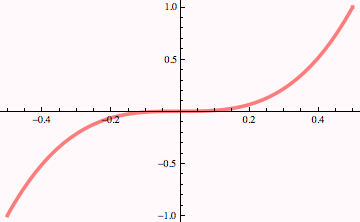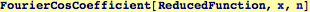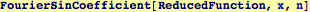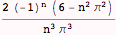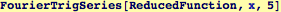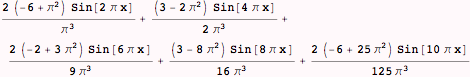Created by Wolfram Mathematica 6.0  (24 October 2007)